# CBSE Solutions for Class 10 English

#### GSEB std 10 science solution for Gujarati check Subject Chapters Wise::

Fill in the blanks :- The concept of grouping elements into triads was given by ……… .

જવાબ : Dobereiner

Fill in the blanks :- The basis for Modern Periodic Table is ……… .

જવાબ : atomic no.

An element A is in group II (group 2) of the periodic table:
(а) What will be the formula of its chloride?
(b) What will be the formula of its oxide?

જવાબ : (a) ACl2
(b) AO.

Why are the elements calcium, strontium and barium named as alkaline earths?

જવાબ : These elements are called alkaline earths because their oxides are alkaline in nature and exist in the earth.

Name the elements present in period 1 of the modern periodic table.

જવાબ : Hydrogen (H) and Helium (He).

Fill in the blanks :- Mendeleev’s basis for the Periodic Table is ……… .

જવાબ : atomic mass

Fill in the blanks :-

(a) Metallic character ……… down the group.
(b) Atomic size ……… along the period.
(c) Electronegative character ……… down the group.

જવાબ : (a) increases
(b) decreases
(c) decreases

Fill in the blanks :-  Isotopes belong to the same ……… in the Periodic Table.

જવાબ : position

Fill in the blanks :- Halogens belong to group ……… of the Periodic Table.

જવાબ : 17

Fill in the blanks :- An element having electronic configuration (2, 8, 2) belongs to the ……… group.

જવાબ : 12th

Fill in the blanks :- Atoms of different elements with the same number of occupied shells are placed in the same ……… .

જવાબ : Period

Fill in the blanks :- Valency of elements ……… and then ……… as we move across the period while it remains the same down the group.

જવાબ : increases, decreases

Fill in the blanks :- Non-metals are located on the ……… side of the Periodic Table.

જવાબ : right

I am the Modern Periodic Table, where elements are arranged on the basis of ……………. I have horizontal rows called ……………. and ……………. vertical columns are called ……………. My first period is very short with ……………. elements and second and third periods contain ……………. elements, fourth and fifth ……………. has ……………. elements. Sixth period has ……………. elements but seventh period is ……………. with space for more elements. 17 group is of ……………., 18 is of ……………. Atomic size of the elements ……………. across the period but ……………. down the group. Metallic character ……………. across the period but non-metallic character ……………. Number of valence electrons ……………. across the period and the valency ……………. but valency of the elements is ……………. in a group. All the properties of elements are the Periodic function of their …………….

જવાબ : atomic number
seven
periods
eighteen
groups
two
eight
eighteen
thirty two
incomplete
halogen
noble gases
decreases
increases
decreases
increases
increases
first increases and then decreases
same
atomic number.

Use Mendeleev’s Periodic Table to predict the formulae for the oxides of the following elements : K, C, Al, Si, Ba

જવાબ : K2O, CO2, Al2O3, SiO2, BaO.

Besides gallium, which other elements have since been discovered that were left by Mendeleev in his periodic table ? (any two)

જવાબ : Scandium and Germanium.

Besides gallium, which two other elements have since been discovered that fill the gaps left by Mendeleev in his periodic table?

જવાબ : Two other elements are scandium (Sc) and germanium (Ge). In their gaps, the elements with names Eka-boron and Eka-silicon were placed.

What were the criteria used by Mendeleev in creating his periodic table?

જવાબ : Mendeleev used atomic masses of the elements as the criteria for creating his periodic table. In this table, the elements were arranged in order of increasing atomic masses.

Which properties remain similar on moving down a group (of Modern Periodic Table) - physical or chemical?

જવાબ : Chemical properties.

Why do all the elements in a group of the Modern Periodic Table have similar chemical properties?

જવાબ : All the elements of a group of the Modern Periodic Tabe have similar chemical properties because they have the same number of valence electrons in their outermost shell.

Define Modern Periodic Law.

જવાબ : Modern Periodic Law states that properties of elements are a periodic function of their atomic number.

How does the electronegativity of elements relate to their metallic or non-metallic character?

જવાબ : Electronegativity of an element is the tendency of its atom to attract electrons towards itself when bonded to the atom of another element. So, the elements that are electronegative, i.e., tend to form bonds by gaining electrons are the non-metals. On the other hand, elements with low or negligible electronegativity are known as metals.

Name two elements whose properties were predicted on the basis of their positions in Mendeleev’s periodic table.

જવાબ : Gallium (Eka-aluminium) and germanium (Eka-silicon).

Write the formulae of chlorides of Eka-silicon and Eka-aluminium, the elements predicted by Mendeleev.

જવાબ : Eka-aluminium represents gallium (Ga) with valency three and Eka-silicon is for germanium (Ge) with valency four. The formulae of their respective chlorides are GaCl3 and GeCl4.

How many elements are there in period 2?

જવાબ : Eight (8).

Name all the elements present in group-17 of the Modern Periodic Table.

જવાબ : F, Cl, Br, I, At.

Name two elements whose atomic weight were corrected on the basis of their position in Mendeleev’s periodic table.

જવાબ : Gold (Au) and platinum (Pt).

Arrange the following elements in the increasing order of their metallic character.
Mg, Ca, K, Ge, Ga

જવાબ : Ge < Ga Mg < Ca < K.

Name the scientist who proposed the Modern Periodic Law.

જવાબ : Henry Moseley, a scientist, proposed the Modern Periodic Law.

What is meant by a group in the periodic table?

જવાબ : Groups are the vertical columns/vertical lines in the periodic table.

What is meant by a period in the periodic table?

જવાબ : Periods are the horizontal rows in the periodic table.

Give the name and electronic configuration of second alkali metal.

જવાબ : The second alkali metal is sodium (Na). Its electronic configuration is 2, 8, 1.

What are Metalloids?

જવાબ : Those elements which show some properties of both metals and non-metals are called metalloids. Examples: Boron, Silicon, Germanium, Arsenic, Antimony, Tellurium and Polonium.

Name three elements whose atomic masses were correct on the basis of their positions in Mendeleev’s periodic table.

જવાબ : Beryllium (Be, group II A)
gold (Au, group II B)
platinum (Pt, group VIII).

Name three elements which behave as metalloids.

જવાબ : The elements are:
arsenic (As)
antimony (Sb)
germanium (Ge).

Name the inert gas which has two electrons in its valence shell.

જવાબ : Helium.

Name the most metallic and most non-metallic element in the Periodic Table.

જવાબ : The most metallic elements is francium (group 1) and most non-metallic element is fluorine (group 17).

In the Modern Periodic Table, which are the metals among the first ten elements?

જવાબ : Lithium (Li), Beryllium (Be) and Boron (B) are the metal elements among first ten elements in the Modern Periodic Table.

Two elements A and B belong to the same period. What is common in them?

જવાબ : They have the same number of shells.

The electronic configuration of an element is 2, 8, 6. Identify the element and name of the family to which it belongs.

જવાબ : The element with configuration 2, 8, 6 (Z = 16) is sulphur. It belongs to the oxygen family.

Calcium, Strontium and Barium form a Dobereiner’s triad. The atomic masses of calcium and Barium are 40 and 137 respectively. Predict the atomic mass of strontium.

જવાબ : According to Dobereiner’s triad, atomic mass of strontium is the arithmetic means of Ca and Ba as strontium lies between Ca and Ba.
Atomic mass of Sr = 40+1372=1772=88.5

The formula of magnesium oxides is MgO. Write the formula of magnesium chloride.

જવાબ : Oxygen is divalent in nature. The valency of magnesium in magnesium oxide is + 2. The formula of magnesium chloride is MgCl2 since chlorine has valency equal to one.

State whether the following statement is true or false?
The valency of an element of group 17 is 7.

જવાબ : This statement is wrong. The number of valence electrons in an element of group 17 = 17 – 10 = 7.
Therefore, the valency of the element = 8 – 7 = 1.

Name two elements whose atomic weight were corrected on the basis of their position in Mendeleev periodic table.

જવાબ : Gold (Au)
Platinum (Pt).

The two isotopes of chlorine have atomic mass 35.4 and 37.4. Should they be placed in separate slots in the modern periodic table?

જવાબ : No, they should be placed in the same slot because the modern periodic table is based on the atomic number of the elements.

List any two properties of the elements belonging to the first group of the modem periodic table.

જવાબ : Elements of first group have one valence electron each in their atoms. All the elements of group 1 have the same valency of 1.

What is the basic difference in the electronic configuration of the elements belonging to group 1 and group 2?

જવાબ : All elements belonging to group 1 have one electron in the valence shell while all elements belonging to group 2 have two electrons in their valence shell.

Element ‘Y’ with atomic number 3 combines with element ‘A’ with atomic number 17. What will be the formula of the compound?

જવાબ : The electronic distribution in elements ‘A’ and ‘Y’ are 2, 1 and 2, 8, 7 respectively. Both have valency equal to 1. The formula of the compound is AY.

Name the property which remains unchanged on descending a group in the periodic table.

જવાબ : Valence electrons.

An element X is in group 13 of the periodic table. What is the formula of its oxide?

જવાબ : X2O3.

Elements ‘X’ and TP belong to groups 1 and 17 of the periodic table respectively. What will be the nature of the bond in the compound XY?

જવાબ : Ionic bond.

Nitrogen (atomic number 7) and phosphorus (atomic number 15) belong to group 15 of the periodic table. Write the electronic configuration of these two elements. Which of these will be more electronegative ? Why ?

જવાબ : Electronic configuration of nitrogen -2,5
Electronic configuration of phosphorus = 2, 8, 5
Nitrogen will be more electronegative because outermost shell is nearer to nucleus and therefore nucleus will attract electrons more strongly. In a group of the periodic table, electron attracting tendency decreases as we move from top to bottom.

(a) Lithium, sodium, potassium are all metals that react with water to liberate hydrogen gas. Is there any similarity in the atoms of these elements ?
(b) Helium is an unreactive gas and neon is a gas of extremely low reactivity. What, if anything, do their atoms have in common ?

જવાબ : (a) Lithium, sodium and potassium all belong to the same group. The atoms of lithium, sodium and potassium all have only one electron in their outermost shells and all of these are metals. All of these react with water to form alkalies.
(b) The atoms of helium and neon have their outermost shells completely filled. Helium has its first shell completely filled, while neon has its first and second shells (K and L) completely filled.

How could the modern periodic table remove various anomalies of Mendeleev’s periodic Table ?

જવાબ : (i) The modern periodic table is based on atomic number, while Mendeleev’s periodic table was based on atomic mass.
(ii) The isotopes of an element have same number of protons (or atomic number). So they are alloted the same position in modern periodic table.
(iii) Cobalt and nickel are placed at 9th and 10th position respectively.
(iv)  Hydrogen has been alloted special position, i.e., it is placed at the top of alkali metals in the first group.

What were the limitations of Newlands’ law of octaves ?

જવાબ : (i) Newlands law of octaves was applicable to the classification of elements upto calcium only. After calcium every eighth element did not possess the properties similar to that of the first element.
(ii) Newlands assumed that only 56 elements existed in nature and no more elements would be discovered in the future. But later on, several new elements were discovered whose properties did not fit into Newlands’ law of Octaves.
(iii) In order to fit elements into his table, Newlands put even two elements together in one slot and that too in the column of unlike elements having very different properties.
For example, the two elements cobalt (Co) and nickel (Ni) were put together in just one slot and that too in the column of elements like fluorine, chlorine and bromine which have very different properties from these elements.
(iv) Iron (Fe) element which resemble elements like cobalt and nickel in properties, was placed far away from these elements.

Compare and contrast the arrangement of elements in Mendeleev’s periodic Table and the Modern Periodic Table.

જવાબ :

 Mendeleev’s periodic table Modern periodic table 1. Elements are arranged in the increasing order of their atomic masses. 1. Elements are arranged in the increasing order of their atomic numbers. 2. There are a total of 7 groups (columns) and 6 periods (rows). 2. There are a total of 18 groups (columns) and 7 periods (rows). 3. Elements having similar properties were placed directly under one another. 3. Elements having the same valence shell are present in the same period while elements having the same number of valence electrons are present in the same group. 4. The position of hydrogen could not be explained. 4. Hydrogen is placed above alkali metals. 5. No distinguishing positions for metals and non-metals. 5. Metals are present at the left hand side of the periodic table whereas non-metals are present at the right hand side.

Which element has

(a) two shells, both of which are completely filled with electrons?

(b) the electronic configuration 2, 8, 2?

(c) a total of three shells, with four electrons in its valence shell?

(d) a total of two shells, with three electrons in its valence shell?

(e) twice as many electrons in its second shell as in its first shell?

જવાબ : (a) Neon has two shells, both of which are completely filled with electrons (2 electrons in K shell and 8 electrons in L shell).

(b) Magnesium has the electronic configuration 2, 8, 2.

(c) Silicon has a total of three shells, with four electrons in its valence shell (2 electrons in K shell, 8 electrons in L shell and 4 electrons in M shell).

(d) Boron has a total of two shells, with three electrons in its valence shell (2 electrons in K shell and 3 electrons in L shell).

(e) Carbon has twice as many electrons in its second shell as in its first shell (2 electrons in K shell and 4 electrons in L shell).

Use Mendeleev’s Periodic Table to predict the formulae for the oxides of the following elements:

K, C, Al, Si, Ba.

જવાબ : K is in group 1. Therefore, the oxide will be K2O.

C is in group 4. Therefore, the oxide will be CO2.

Al is in group 3. Therefore, the oxide will be Al2O3.

Si is in group 4. Therefore, the oxide will be SiO2.

Ba is in group 2. Therefore, the oxide will be BaO.

What were the limitations of Newlands’ Law of Octaves?

જવાબ : Limitations of Newlands’ law of octaves:

(i) It was not applicable throughout the arrangements. It was applicable up to calcium only. The properties of the elements listed after calcium showed no resemblance to the properties of the elements above them.

(ii) Those elements that were discovered after Newlands’ octaves did not follow the law of octaves.

(iii) The position of cobalt and nickel in the group of the elements (F, Cl) of different properties could not be explained.

(iv) Placing of iron far away from cobalt and nickel, which have similar properties as iron, could also not be explained.

In the Modern periodic table, calcium (atomic number 20) is surrounded by elements with atomic numbers 12, 19, 21 and 38. Which of these have physical and chemical properties resembling calcium?

જવાબ : The atomic number of calcium is 20, so its electronic configuration is 2, 8, 8, 2. Thus, calcium has 2 valence electrons (in its outermost shell). Now, the element which has 2 valence electrons, will have physical and chemical properties resembling to that of calcium. The electronic configuration of element having atomic number 12 is 2, 8, 2. It has 2 valence electrons just like calcium. So, the element having atomic number 12 will have physical and chemical properties resembling that of calcium.

Compare and contrast the arrangement of elements in Mendeleev’s Periodic Table and the Modern Periodic Table.

જવાબ :

 Mendeleev’s Periodic Table Modern Periodic Table (i) Elements are arranged in the order of increasing atomic mass. (i) Elements are arranged in the order of increasing atomic number. (ii) There are nine vertical columns called groups. (ii) There are eighteen vertical columns called groups. (iii) There is no place for noble gases. (iii) Noble gases are placed on the right hand side of the table. (iv) There is no place for isotopes. (iv) Isotope are kept at the same place because their atomic number is same. (v) Transition elements are placed together in group VIII. (v) Transition elements are placed in the middle of the long period (Group 3 to 12).

How does the electronic configuration of an atom relate to its position in the Modern Periodic Table ?

જવાબ : Modern periodic table is based on the atomic number and atomic number is directly related to the electronic configuration. One can find the group number and period number of an element on the basis of electronic configuration. For example, if an element has 1 or 2 electrons in its outermost shell, then it would belong to group 1 or group 2. And if it has 3 or more electrons in its outermost shell, then it would belong to group 10 4- the number of electrons in the outermost shell.

All the alkali metals have one electron in their outermost shell, so they are placed in group 1. Thus, all the group 2 elements have 2 electrons in their outermost shell. In group 15 elements, there are 5 electrons in their outermost shell. Similarly, the number of shells in an element indicates its period number. For example, the atomic number of magnesium is 12 and its electronic configuration is 2, 8, 2. Thus it is an element of 3rd period.

Ria and Ram are students of class X. Ria is very organised and systematic. The teachers love her. She earns a great respect in the class whereas Ram is unorganised and always faces a lot of problem in handling life situations.
(i) In your opinion how does organisation help in daily life?
(ii) How can you relate the above fact with the chapter classification of elements. How does classification of elements help us in studying them properly?

જવાબ : (i) Organised approach always make the work easier and quicker. Hence, classification makes the things easier.

(ii) Organised approach in classification of elements make the study easier and we get a group of elements with similar properties.
By this approach we can study the elements easily which lies in a group with similar chemical properties.

Atomic number of a few elements are give below:
10, 20, 7, 14
(a) Identify the elements.
(b) Identify the Group number of these elements in the Periodic Table.
(c) Identify the Periods of these elements in the Periodic Table.
(d) What would be the electronic configuration for each of these elements?
(e) Determine the valency of these elements.  [NCERT Exemplar]

જવાબ : (a) The element are: Neon (Z = 10), Calcium (Z = 20), Nitrogen (Z = 7) and Silicon (Z = 14).
(b) Group number: Neon (18), Calcium (2), Nitrogen (15), Silicon (14).
(c) Periods: Neon (2), Calcium (4), Fluorine (2), Silicon (3).
(d) Electronic Configuration: Neon (2, 8); Calcium (2, 8, 8, 2); Nitrogen (2, 5); Silicon (2, 8, 4).
(e) Valency: Neon (zero); Calcium (2); Nitrogen (3); Silicon (4).

(a) Why do we classify elements?
(b) What were the two criteria used by Mendeleev in creating his Periodic Table?
(c) Why did Mendeleev leave some gaps in his Periodic Table?
(d) In Mendeleev’s Periodic Table, why was there no mention of Noble gases like Helium, Neon and Argon?
(e) Would you place the two isotopes of chlorine, Cl-35 and Cl-37 in different slots because of their different atomic masses or in the same slot because their chemical properties are the same? Justify your answer.

જવાબ : (a) As different elements were being discovered, scientists gathered more information about the properties of these elements. It was observed that it was difficult to organise all the information or properties of these elements. So scientists started discovering some pattern in their properties to classify all the known elements to make their study easier.

(b) Atomic mass and similarity of chemical properties (compounds formed by elements with oxygen and hydrogen) were the two criteria used by Mendeleev in his Periodic Table.

(c) Mendeleev left some gaps in his Periodic Table as he predicted the existence of some elements that had not been discovered at that time.

(d) Noble gases like helium, neon, argon, etc. were not mentioned in Mendeleev’s Periodic Table because these gases were discovered later as they are very inert and present in extremely low concentrations in our atmosphere. After the discovery of noble gases they could be placed in a new group without disturbing the existing order of the Periodic Table.

(e) The Modem Periodic Table states that properties of elements are a periodic function of their atomic numbers, since Cl-35 and Cl-37 isotopes have the same atomic number (17), hence they will have same chemical properties even though their atomic masses are different. So, they should be placed in the same slot of the periodic table.

An element X (atomic number 17) reacts with an element Y (atomic number 20) to form a divalent halide.
(a) Where in the periodic table are elements X and Y placed?
(b) Classify X and Y as metal, non-metal or metalloid.
(c) What will be the nature of oxide of element Y? Identify the nature of bonding in the compound formed.
(d) Draw the electron dot structure of the divalent halide.   [NCERT Exemplar]

જવાબ : (a) X belongs to Group 17 and 3rd Period.
Y belongs to Group 2 and 4th Period.
(b) X-non-metal and Y-metal.
(c) Basic oxide; Ionic bonding.
(d)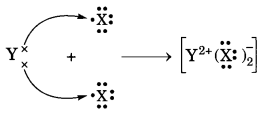​​​​​​

An element X which is a yellow solid at room temperature shows catenation and allotropy. X forms two oxides which are also formed during the thermal decomposition of ferrous sulphate crystals and are the major air pollutants.
(a) Identify the element X
(b) Write the electronic configuration of X
(c) Write the balanced chemical equation for the thermal decomposition of ferrous sulphate crystals.
(d) What would be the nature (acidic/basic) of oxides formed?
(e) Locate the position of the element in the Modern Periodic Table.  [NCERT Exemplar]

જવાબ : (a) The element X which is a yellow solid at room temperature and shows catenation and allotropy is sulphur (S).

(b) The atomic number of sulphur is 16. Therefore, its electronic configuration is 2, 8, 6.

(c)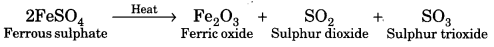Both SO2 and SO3 are major air pollutants.

(d) Since sulphur is a non-metal, therefore, both SO2 and SO3 are acidic oxides since they dissolve in water to form the corresponding acids.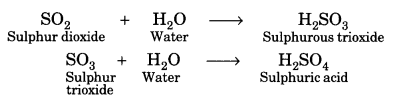(e) Since sulphur contains six valence electrons, therefore, it lies in group 6 + 10 = 16. Further, since atomic number of sulphur (S) is 16, it lies in the 3rd period.

What is meant by ‘group’ in the modern periodic table? How do the following change on moving from top to bottom in a group?
(i) Number of valence electrons
(ii) Number of occupied shells
(iii) Size of atoms
(iv) Metallic character of elements
(v) Effective nuclear change experienced by valence electrons.  [CBSE 2014]

જવાબ : The vertical columns in a periodic table are called groups.
(i) All the elements of a group have the same number of valence electrons.
(ii) On moving down in a group the number of occupied (filled) shells increases gradually.
(iii) On going down in a group the size of atoms increases because a new shell of electrons is added to the atoms at every step.
(iv) On going down in a group the metallic character of elements increases.
(v) On moving down in a group, one more electron shell is added at every stage and size of the atom increases. Thus valence electrons move more and more away from the nucleus and hold of the nucleus or nuclear charge on valence electrons decreases.

Atomic number of an element is 16. Predict
(i) the number of valence electrons in its atom
(ii) its valency
(iii) its group number
(iv) whether it is a metal or a non-metal
(v) the nature of oxide formed by it.
(vi) the formula of the chloride   [CBSE2011]

જવાબ : The element with atomic number Z = 16 is sulphur. The electronic configuration of the element is 2, 8, 6.
(i) The number of valence electrons is 6
(ii) The valency of the element is 2 (8 – 6 = 2)
(iii) The group number of the element is 16.
(iv) The element is a non-metal.
(v) Since the element is non-metal, its oxide is acidic in nature. Actually, two oxides are formed. These are SO2 and SO3. Both are of acidic nature.
(vi) The formula of the chloride is SCl2.

The element Li, Na and K, each having one valence electron, are in period 2, 3 and 4 respectively of the Modem Periodic Table.
(a) In which group of the periodic table should they be?
(b) Which one of them is least reactive?
(c) Which one of them has the largest atomic radius? Give reason to justify your answer in each case. [CBSE 2013]

જવાબ : (a) Since the elements have one valence electron, they are placed in group 1 (Alkali metals) in the order Li (period = 2); Na (period = 3); K (period = 4).

(b) Since the reactivity of the elements increases down a group, the element Li is the least reactive chemically.

(c) Since the atomic size increases down a group, the element K has the large atomic size or atomic radius out of these elements.

Two elements X and Y belong to group 1 and 2 respectively in the same period of periodic table. Compare them with respect to:   [CBSE 2011]
(i) the number of valence electrons in their atoms
(ii) their valencies
(iii) metallic character
(iv) the sizes of their atoms
(v) the formulae of their oxides
(vi) the formulae of their chlorides.

જવાબ : X and Y belong to same period.

• X belongs to group ‘1’.
• Y belongs to group ‘2’.
(i) Valence electron in X is 1 whereas valence electrons in Y are 2.
(ii) The valency of X is 1 whereas valency of Y is 2.
(iii) X is more metallic than Y because metallic character decreases on moving from left to right in a period.
(iv) The size of X is more than Y because size of the atom decreases on moving from left to right in a period.
(v) Oxide of X = X2O,   Oxide of Y = YO
(vi) Chloride of X = XCl,   Chloride of Y = YCl2

Two elements X and Y have atomic number 12 and 17 respectively.
(i) Write the electronic configuration of both.
(ii) Which type of bond will they form?
(iii) Write the formula of the compound formed by their combination (in terms of X and Y).

જવાબ : (i) The electronic configuration of element X (Z = 12) = 2, 8, 2
The electronic configuration of element Y (Z = 17) = 2, 8, 7

(ii) The bond formed will be of ionic nature. One atom of X will transfer 1 electron each to two atoms of Y.

(iii) The formula of the compound is: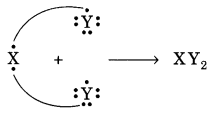The electronic configuration of an element X is: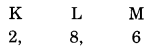(i) What is the group number of element X in the periodic table?
(ii) What is the period number of element X in the periodic table?
(iii) What is the number of valence electrons in an atom of X?
(iv) What is the valency of X?

જવાબ : (i) From the above given electronic configuration we find that element X has 6 valence electrons in the outermost shell), so the group number of element X in the periodic table is 6 + 10 = 16.

(ii) Element X has 3 electron shells (K, L and M) in its atom, so the period number of X is 3. That is, X belongs to 3rd period of the periodic table.

(iii) Element X has 6 valence electrons.

(iv) Element X has 6 valence electrons so it needs 2 more electrons to complete its octet (8 electrons in valence shell) and become stable. Thus, the valency of element X is 2.

How many groups and periods are there in the modern periodic table? How do the atomic size and metallic character of elements vary as we move: (a) down a group and (b) from left to right in a period.

જવાબ : Number of groups is 18 and number of periods in the modern periodic table is 7.
(a) All the elements in a group have the same valency. On going down in a group, the atomic size increases because a new shell of electrons is added to the atoms at every step.

(b) On moving from left to right in the periodic table, the valency of the elements first increases from 1 to 4 and then decreases to zero. On moving from left to right in a period, the metallic character of elements decrease because on moving from left to right in a period, the electropositive character of elements decrease.

Na, Mg and Al are the elements of the same period of Modern Periodic Table having one, two and three valence electrons respectively. Which of these elements (i) has the largest atomic radius, (ii) is least reactive? Justify your answer stating reason for each case.   [Delhi 2015]

જવાબ :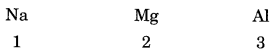(1) Na has the largest atomic radius because on moving from left to right in the periodic table, the atomic radius decreases due to increase in positive charge on the nucleus which pulls the outermost electrons more close to the nucleus and the size of atom decreases.

(2) Al is least reactive because on moving from left to right in the periodic table the nuclear charge increases and the valence electrons are pulled in more close to the nucleus. Therefore, the tendency to lose electrons decreases and hence reactivity decreases.

Write two reasons responsible for the late discovery of noble gases

જવાબ :

1. Noble gas elements were not present in earth crust as minerals like other elements and were present in air to a very small extent.
2. Their atoms have stable electronic configuration of their outermost shells also called valence shells. (2 in case of He and 8 for other elements). They do not combine with atoms of other elements.
That is why, noble gas elements were discovered at a later stage.

Explain why:
(i) All the elements in a group have similar chemical properties.
(ii) All the elements in a period have different chemical properties.

જવાબ : (i) All the elements in a particular group have similar outer shell electronic configuration. Since chemical properties of an element are determined mainly by the outer shell configuration, all the elements in a group have similar chemical properties.

(ii) All the elements in a period have different valence shell electronic configuration because they have different number of electrons in the valence shell. Hence, the elements in a period have different chemical properties.

How does the electronic configuration of an atom of an element relate to its position in the modem periodic table? Explain with one example.

જવાબ : The electronic configuration of an atom of an element gives its position in the modern periodic table.
(i) The ‘period number’ of an element is equal to the number of electron shells in its atom.
(ii)

• The group number of an element having upto two valence electrons is equal to the number of valence electrons.
• The group number of an element having more than 2 valence electrons is equal to the number of valence electrons plus 10.
Example: If the electronic configuration of an element is 2, 8, 7.
Then its period number is 3 as it has three electrons shells.
Its group number is 17 as it has 7 valence electrons. (∵ Group no. = 7 + 10 = 17)

Explain clearly, why atomic number of an element is most important to the chemist than its relative atomic mass.

જવાબ : Atomic number corresponds to the number of electron in an atom or it reflects the electronic configuration of the element. The elements having similar electronic configurations can then be placed together in the same group, it helps in the systematic classification of elements.

On the other hand, the atomic mass of an element does not provide the electronic configuration of an element, so atomic number is more fundamental property for classification of elements.

If an element X is placed in group 14, what will be the formula and the nature of bonding of its chloride?

જવાબ : Since element “X’ is placed in group 14, therefore, its valency is 14 – 10 = 4. Further, since it is difficult to either lose all the four valence electrons or gain four more electrons, therefore, it prefers to share these four electrons to acquire the stable electronic configuration of the nearest inert gas. Its formula will be XCl4. Thus, the nature of the chloride of element ‘X’ is covalent.

“Hydrogen occupies a unique position in the Modern Periodic Table”. Justify the statement

જવાબ : The position of the element hydrogen is still not clear even in the Modern Periodic Table.

In electronic configuration, it resembles alkali metals of group 1. All of them have only one electron in the valence shell. Actually, hydrogen has only one shell (K-shell) which has one electron.

In characteristics, it resembles halogens of group 17. For example, like halogens hydrogen is a non-metal and diatomic as well. It has been therefore, decided to assign hydrogen a unique position in the Modern or Long Form Periodic Table. It is placed at the top in group 1 of alkali metals. However, it is not a member of that group.

### There are No Content Availble For this Chapter

 Column I Column II (i) Most electronegative element A. Nitrogen (ii) Group 16 element with largest atomic radius B. Polonium (iii) Group 14 element with smallest atomic radius C. Boron (iv) Group 13 element which is semiconductor D. Carbon (v) ‘ Group 15 element which can form triple bond E. Fluorine

જવાબ :

(i) E
(ii) B
(iii) D
(iv) C
(v) A

 a. Highly reactive and soft metal which imparts yellow colour when subjected to flame and is kept under kerosene. Astatine b. The first element of second Period. Iron c. An element which is used in making fluorescent bulbs and is the second member of Group 18 in the Modern Periodic Table. Lithium d. An radioactive element which is the last member of halogen family. Borone e. Metal which is an important constituent of steel and forms rust when exposed to moist air. Neon f. The first metalloid in Modem Periodic Table whose fibres are used in making bullet-proof vests. Sodium

જવાબ :

a. Sodium
b. Lithium
c. Neon
d. Astatine
e. Iron
f. Boron

 a. An element with atomic number 12. Tin b. Metal used in making cans and member of Group 14. Iodine c. lustrous non-metal which has 7 electrons in its outermost shell. Magnesium

જવાબ :

a.  Magnesium
b. Tin
c. Iodine

The table given below shows a part of the periodic table.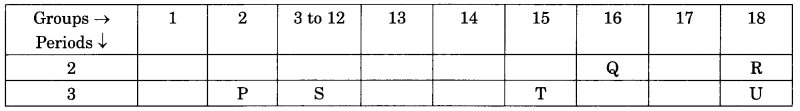Column I Column II (a) P (P) P (b) Q (q) Mg (c) R (r) Ne (d) T (s) O (e) U (t) Ar

જવાબ :

 Column I Column II (a) P (q) Mg (b) Q (s) O (c) R (r) Ne (d) T (P) P (e) U (t) Ar

 Column I Column II (a) F (p) Metallic oxide (b) Na (q) Noble gas (c) Ne (r) group 1, alkali metal (d) Na2O (s) Halogen, group-17

જવાબ :

 Column I Column II (a) F (s) Halogen, group-17 (b) Na (r) group 1, alkali metal (c) Ne (q) Noble gas (d) Na2O (p) Metallic oxide

 Column I Column II (a) Highest electronegativity (P) Caesium (b) Highest electron affinity (q) Ruthenium (c) Highest electropositivity (r) Fluorine (d) Highest oxidation number (s) Chlorine

જવાબ :

 Column I Column II (a) Highest electronegativity (r) Fluorine (b) Highest electron affinity (s) Chlorine (c) Highest electropositivity (P) Caesium (d) Highest oxidation number (q) Ruthenium